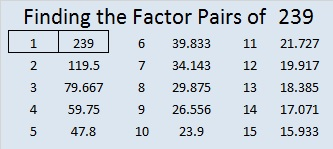# 239 and Level 2

• 239 is a prime number.
• Prime factorization: 239 is prime.
• The exponent of prime number 239 is 1. Adding 1 to that exponent we get (1 + 1) = 2. Therefore 239 has exactly 2 factors.
• Factors of 239: 1, 239
• Factor pairs: 239 = 1 x 239
• 239 has no square factors so its square root cannot be simplified. √239 ≈ 15.4596How do we know that 239 is a prime number? If 239 were not a prime number, then it would be divisible by at least one prime number less than or equal to √239 ≈ 15.4596. Since 239 cannot be divided evenly by 2, 3, 5, 7, 11, or 13, we know that 239 is a prime number.Print the puzzles or type the factors on this excel file: 12 Factors 2014-09-22This site uses Akismet to reduce spam. Learn how your comment data is processed.